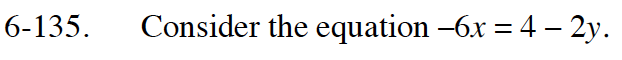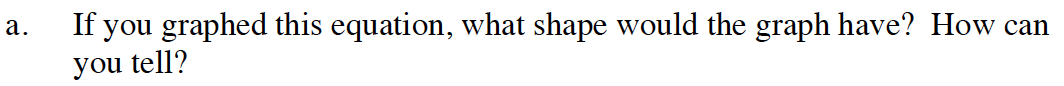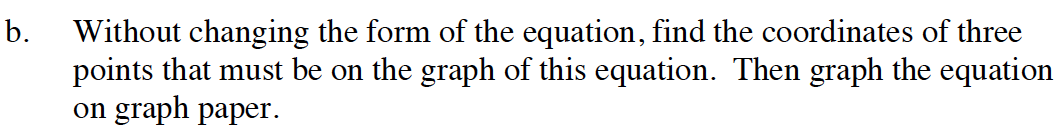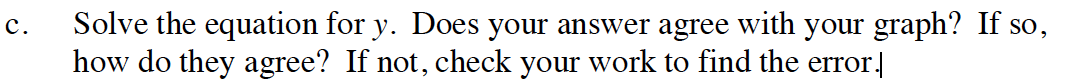Home > GB8I > Chapter cc46 > Lesson cc46.4.2 > Problem6-135

6-135.
1. Consider the equation −6x = 4 − 2y. Homework Help ✎

1. If you graphed this equation, what shape would the graph have? How can you tell?

2. Without changing the form of the equation, find the coordinates of three points that must be on the graph of this equation. Then graph the equation on graph paper.

3. Solve the equation for y. Does your answer agree with your graph? If so, how do they agree? If not, check your work to find the error.If there's an x and a y, and there are no exponents like x², what kind of graph does the equation make?
Remember that this equation could be written in y = mx + b form.Make a table and substitute values for x and y until you have enough points to graph the line.Refer to problems (a) and (b) for help with this problem.

Solve for y:
−6x − 4 = −2y
6x + 4 = 2y

3x + 2 = y
Yes, they both have the same starting value (2) and growth (3).